A wire is broken in four equal parts. A packet is formed by keeping the four wires together. The resistance of the packet in comparison to the resistance of the wire will be

(1) Equal

(2) One fourth

(3) One eight

(4) $\frac{1}{16}th$

High Yielding Test Series + Question Bank - NEET 2020

Difficulty Level:

Four resistances are connected in a circuit in the given figure. The electric current flowing through 4 ohm and 6 ohm resistance is respectively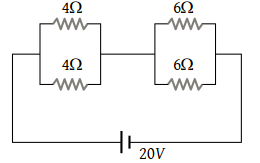(1) 2 amp and 4 amp

(2) 1 amp and 2 amp

(3) 1 amp and 1 amp

(4) 2 amp and 2 amp

High Yielding Test Series + Question Bank - NEET 2020

Difficulty Level:

An infinite sequence of resistance is shown in the figure. The resultant resistance between A and B will be, when R1 = 1 ohm and R2 = 2 ohm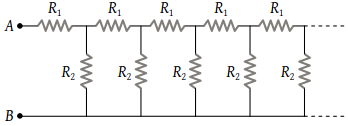(1) Infinity

(2) 1 Ω

(3) 2 Ω

(4) 1.5 Ω

High Yielding Test Series + Question Bank - NEET 2020

Difficulty Level:

In the figure, the value of resistors to be connected between C and D so that the resistance of the entire circuit between A and B does not change with the number of elementary sets used is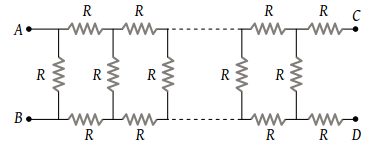(1) R

(2) $R\left(\sqrt{3}-1\right)$

(3) 3 R

(4) $R\left(\sqrt{3}+1\right)$

Concept Questions :-

Combination of resistors
High Yielding Test Series + Question Bank - NEET 2020

Difficulty Level:

In the figure shown, the total resistance between A and B is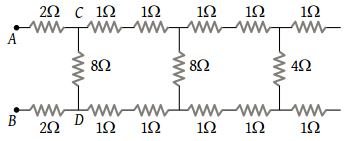(1) 12 Ω

(2) 4 Ω

(3) 6 Ω

(4) 8 Ω

Concept Questions :-

Combination of resistors
High Yielding Test Series + Question Bank - NEET 2020

Difficulty Level:

The current from the battery in circuit diagram shown is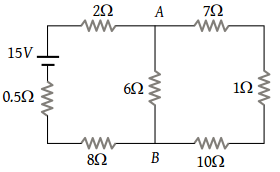(1) 1 A

(2) 2 A

(3) 1.5 A

(4) 3 A

Concept Questions :-

Combination of resistors
High Yielding Test Series + Question Bank - NEET 2020

Difficulty Level:

In the given figure, when key K is opened, the reading of the ammeter A will be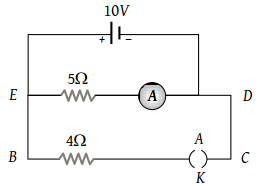(1) 50 A

(2) 2 A

(3) 0.5 A

(4) $\frac{10}{9}A$

Concept Questions :-

Combination of resistors
High Yielding Test Series + Question Bank - NEET 2020

Difficulty Level:

In the given circuit, the potential of the point E is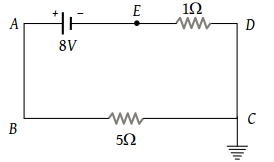(1) Zero

(2) –8 V

(3) –4/3 V

(4) 4/3 V

Concept Questions :-

Kirchoff's voltage law
High Yielding Test Series + Question Bank - NEET 2020

Difficulty Level:

If a resistance R2 is connected in parallel with the resistance R in the circuit shown, then possible value of current through R and the possible value of R2 will be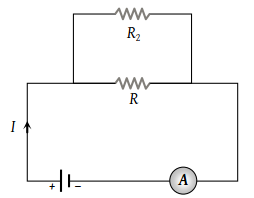(1) $\frac{I}{3},\text{\hspace{0.17em}}R$

(2) $I,\text{\hspace{0.17em}}2R$

(3) $\frac{I}{3},\text{\hspace{0.17em}}R$

(4) $\frac{I}{2},\text{\hspace{0.17em}}R$

Concept Questions :-

Kirchoff's voltage law
High Yielding Test Series + Question Bank - NEET 2020

Difficulty Level:

A battery of e.m.f. 10 V is connected to resistance as shown in figure. The potential difference ${V}_{A}-{V}_{B}$  between the points A and B is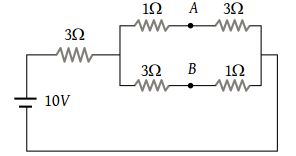(1) –2 V

(2) 2 V

(3) 5 V

(4) $\frac{20}{11}V$

Concept Questions :-

Combination of resistors